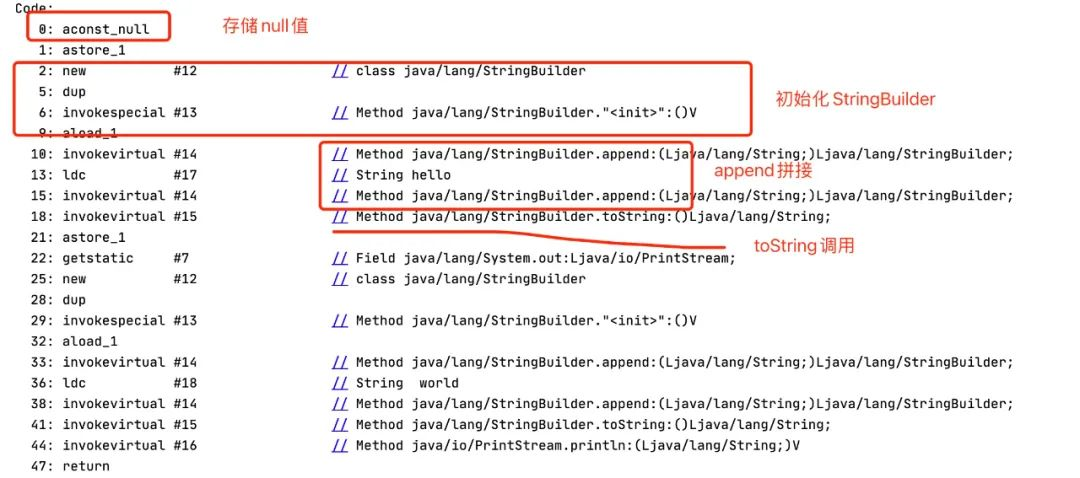# String拼接出现null？你看到的分析可是错的

0收藏

String类型真是个神奇的存在，动不动就会出现一些迷惑人的错误。今天看到一篇文中提到当String的值为null时，进行字符串相加拼接，会出现把null当做字符串拼接的现象。

``````String s = null;
s = s + "hello";
System.out.println(s + " world");``````

``````s + " world" 等价于 s = String.valueOf(s)+"word";
``````

``````public static String valueOf(Object obj) {
return (obj == null) ? "null" : obj.toString();
}``````

Java编译器的优化

``````String a = "Hello ";
String b = "World";
System.out.println(a + b);``````

``````StringBuilder sb = new StringBuilder();
sb.append("Hello ");
sb.append("World");
String result = sb.toString();
System.out.println(result);``````

``````StringBuilder sb = new StringBuilder(null);
sb.append("hello");
sb.append(" world");
String result = sb.toString();
System.out.println(result);``````

``````public AbstractStringBuilder append(String str) {
if (str == null)
return appendNull();
int len = str.length();
ensureCapacityInternal(count + len);
str.getChars(0, len, value, count);
count += len;
return this;
}``````

``````private AbstractStringBuilder appendNull() {
int c = count;
ensureCapacityInternal(c + 4);
final char[] value = this.value;
value[c++] = 'n';
value[c++] = 'u';
value[c++] = 'l';
value[c++] = 'l';
count = c;
return this;
}````````````String s = null;
System.out.println(s);``````

``````public void print(String s) {
if (s == null) {
s = "null";
}
write(s);
}``````

``````Object s = null;
String s1 = String.valueOf(s);
System.out.println(s1);``````

``````Integer i = null;
System.out.println(i);``````

``````public void println(Object x) {
String s = String.valueOf(x);
synchronized (this) {
print(s);
newLine();
}
}``````帖子
视频
声望
粉丝
最近发布
社区精华内容